# Application on shallow Brussels Sand

The Brussels Sand Member is part of the Lower North Sea Group. The top depth of the Brussels Sand reaches depths of up to 1150 m (Figure 1a) and thicknesses up to about 230 m (Figure 1b). These depths are associated with maximum temperatures of 45°C (Figure 1c). Additionally, high permeabilities have been measured with averages of over 150 mD (Figure 1d). These aspects give the Brussels Sand good potential for shallow geothermal production.

 a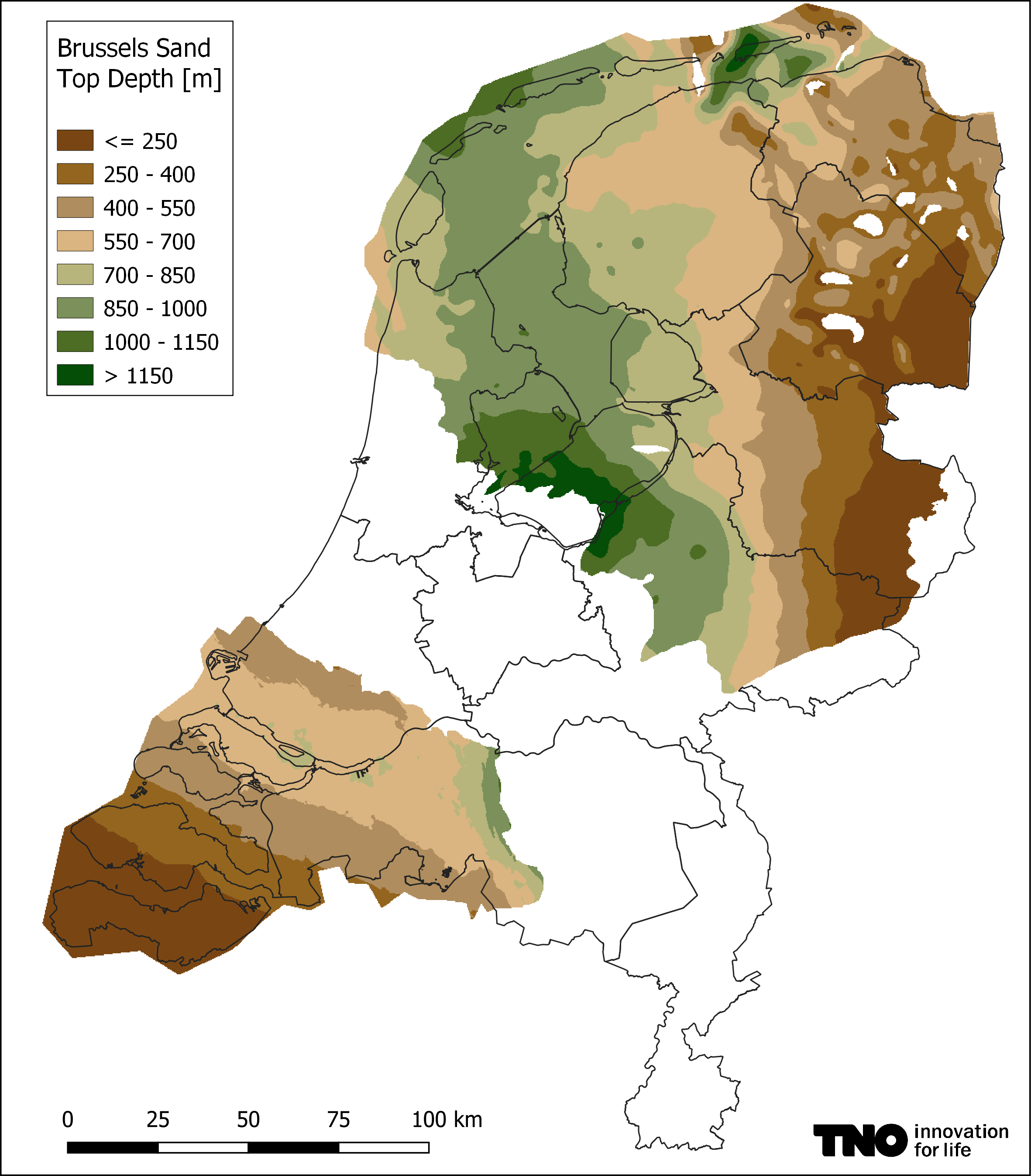b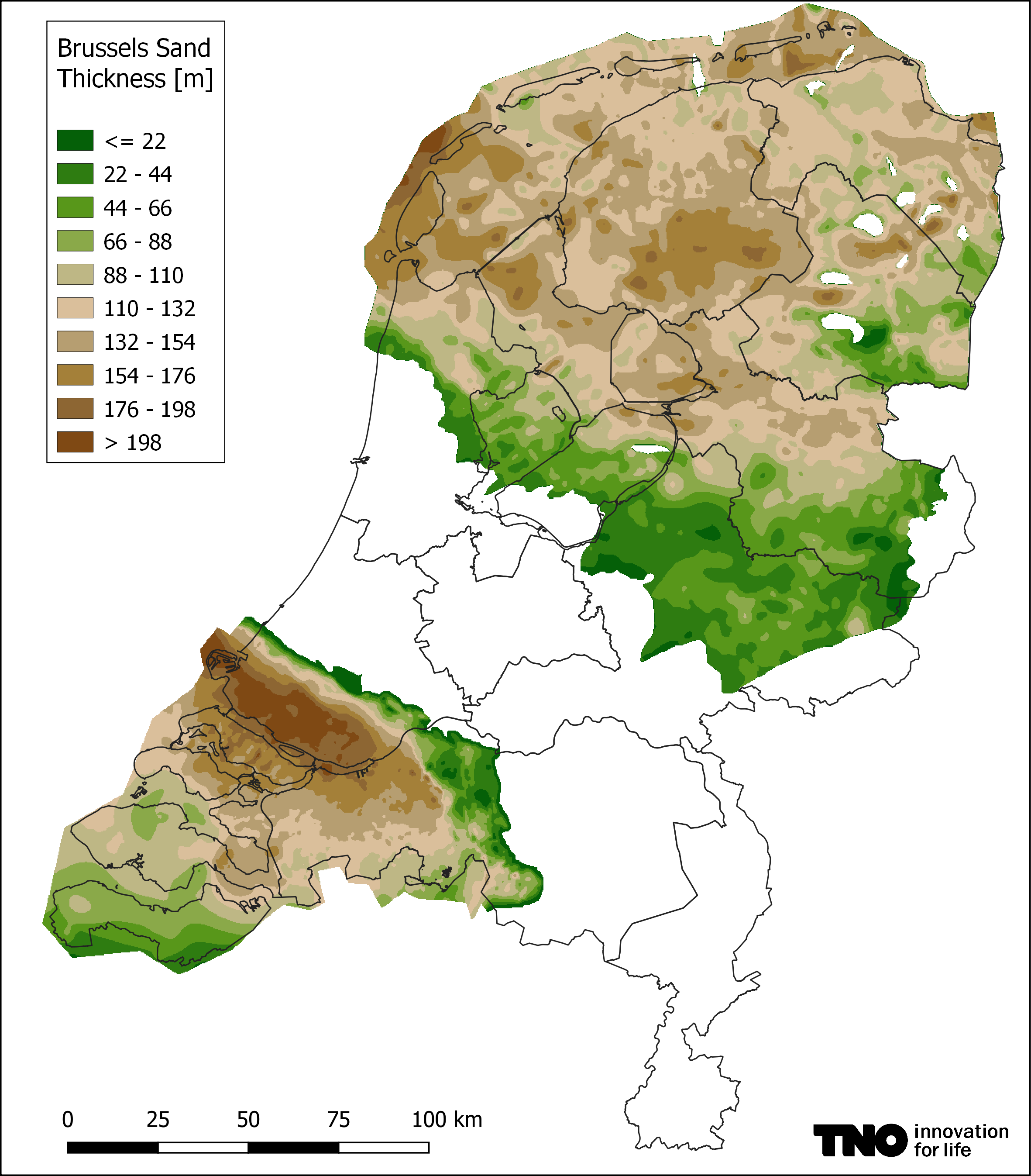cd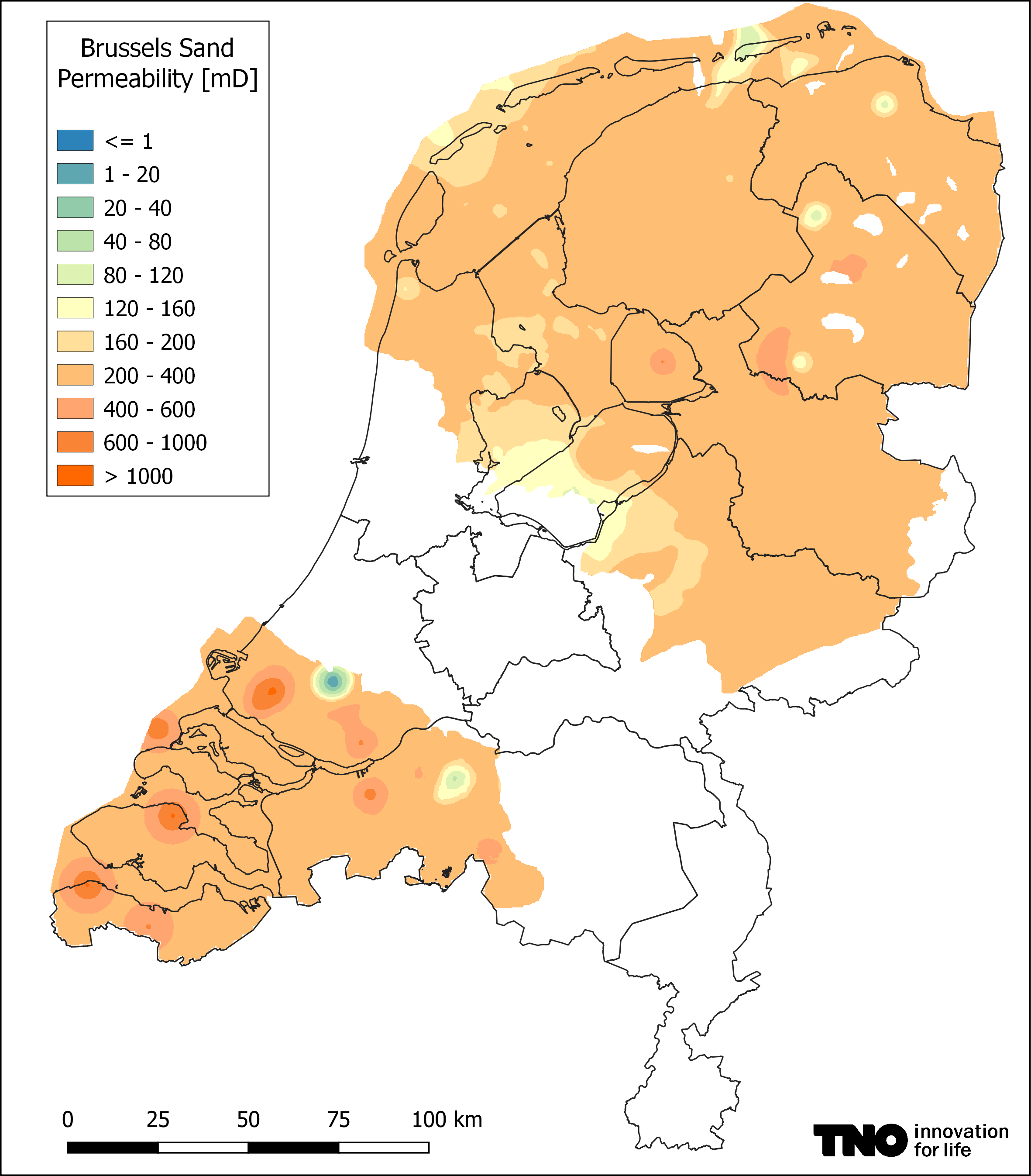Figure 1. Brussels Sand a) top depth, b) thickness, c) temperature at the middle of the aquifer and d) permeability.

## Heat pump concept

The heat pump concept of the standard ThermoGIS method does not take the heat network into account and the COP depends only on the doublet. Therefore, a constant COP of 4 has been used in this standard ThermoGIS method. The adapted heat pump concept does take the heat network into account and the COP of the heat pump is being calculated based on the heat network inlet temperature (i.e. the outlet temperature of the heat exchanger/heat pump to the heat network) and the re-injection temperature of the brine back into the reservoir. This new method enables lower re-injection temperatures than specified by the heat network outlet temperature (i.e. the inlet temperature to the heat exchanger/heat pump from the heat network). By choosing the lowest possible re-injection temperature (till 5°C) the geothermal potential can be maximized. In this scenario, for the Brussels Sand, we assumed a minimum production temperature of 25°C, which was 20°C in the standard ThermoGIS method. For the heat pump concept, we assumed a cooling temperature of 20°C, a heat network inlet temperature of 60°C and a heat network outlet temperature of 40°C.

## Well design

The well design has also been adapted: due to restrictions during the drilling of deviated wells only a limited step-out can be reached at shallow depths. Therefore, it might be possible that for shallow wells (< 1 km) a horizontal well part is necessary in order to obtain the desired distance between injector and producer. The current (standard) implementation of ThermoGIS does not take into account horizontal wells. In order to calculate the length of the well more accurately we added an extra factor: the step-out factor. The step-out factor is the ratio between the True Vertical Depth (TVD) and the step-out (maximum horizontal distance of inclined part of the well) (Figure 2).

stepout factor = TVD / stepout

In this calculation we used a step-out factor of 2. This means that at a depth of 600 m, the step-out factor is 300 m and the distance between producer and injector is 600 m. For example, to obtain a desired distance of 1500 m there must be an addition of 450 m of horizontal well part per well. This gives a more realistic estimation of the well length for shallow wells compared to the calculation method for deep wells. For the perforations the horizontal part is not included, and the same assumptions are taken as for deep wells.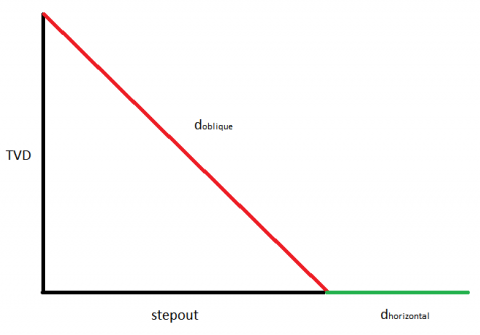Figure 2. Schematic overview in depth of a deviated well with a horizontal well part. In this example the True Vertical Depth (TVD) : step-out ratio (=step-out factor) is 1.

## Results

The standard ThermoGIS results demonstrate that a power of at most 5.6 MWth can be produced (including heat pump) (Figure 3b). The adapted heat pump concept results in an overall small increase of the power values with a maximum of 6.3 MWth (Figure 3c). In the north-northwest (Flevoland, IJsselmeer, Friesland and the Waddeneilanden) and west-southwest (South-Holland and the northwestern part of North-Brabant) high power values are being reached and the Brussels Sand is potentially suitable for shallow geothermal production. In the southwest (Zeeuwse eilanden) and east-northeast (Drenthe) the estimated geothermal power is too low for geothermal production. As these areas do have high transmissivities (Figure 3a) and a low depth interval (Figure 1a) these areas could be suitable for heat storage. The influence of the new well design and the introduction of the step-out factor on the power values is low (Figure 3d).

 a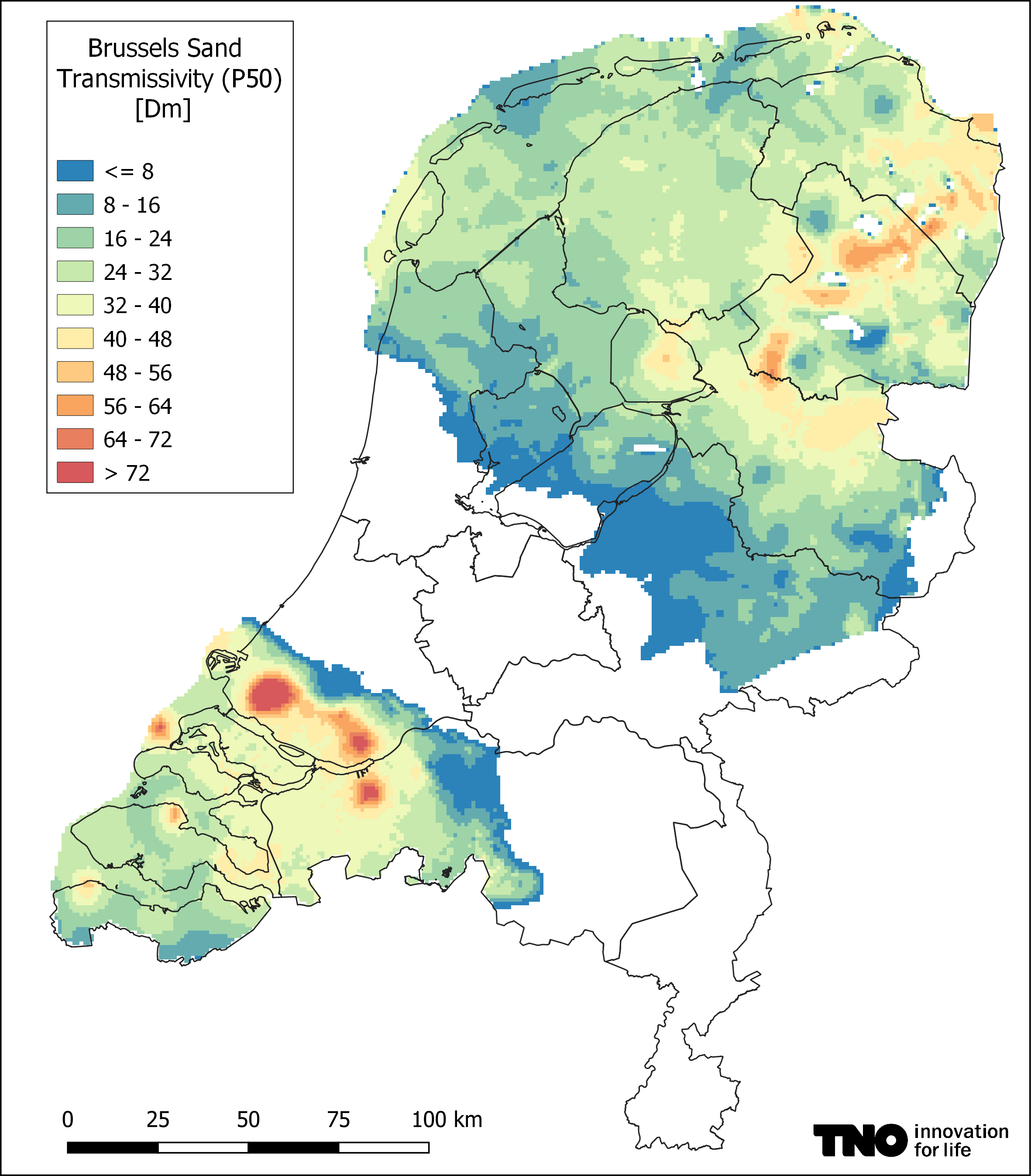b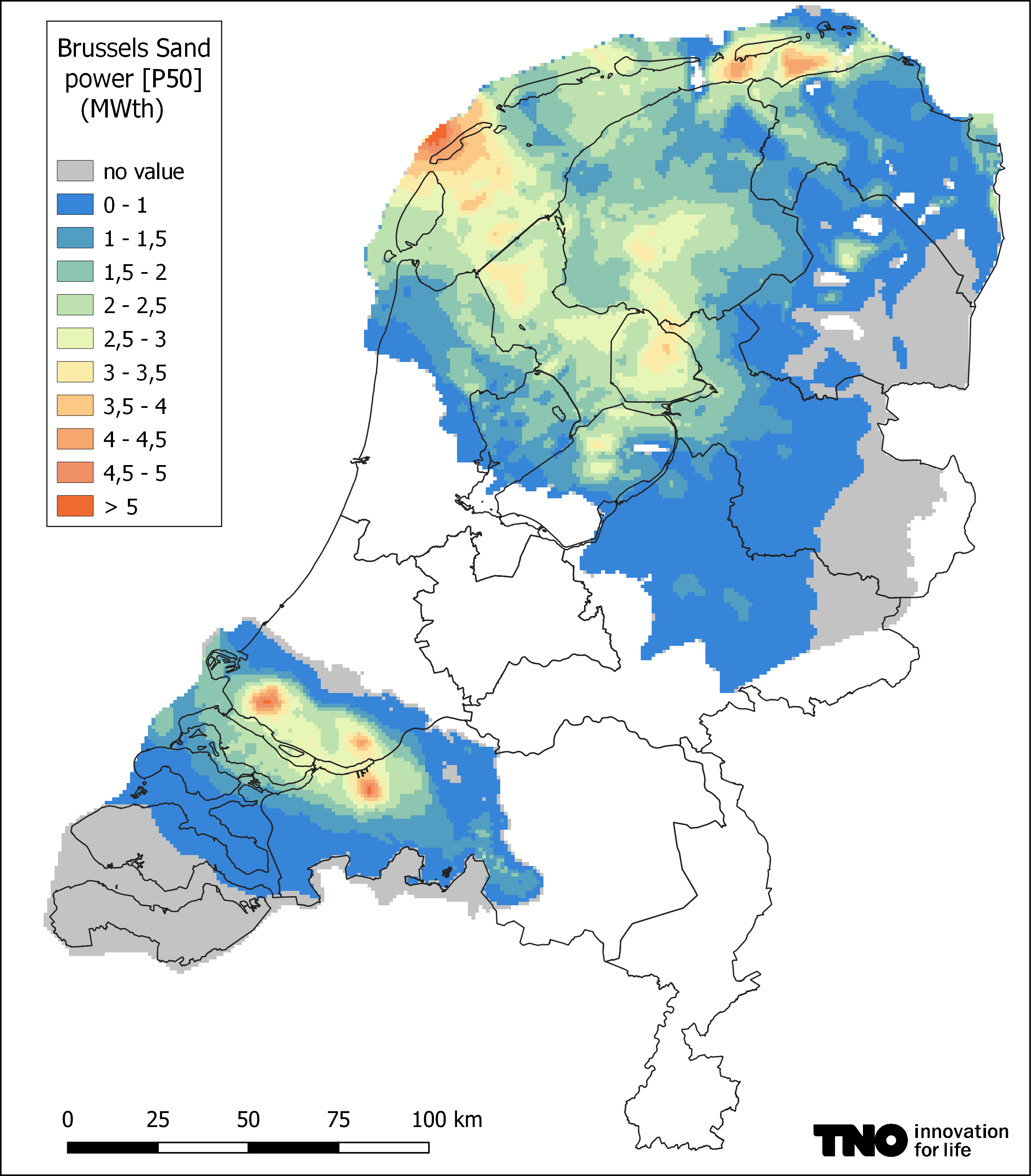c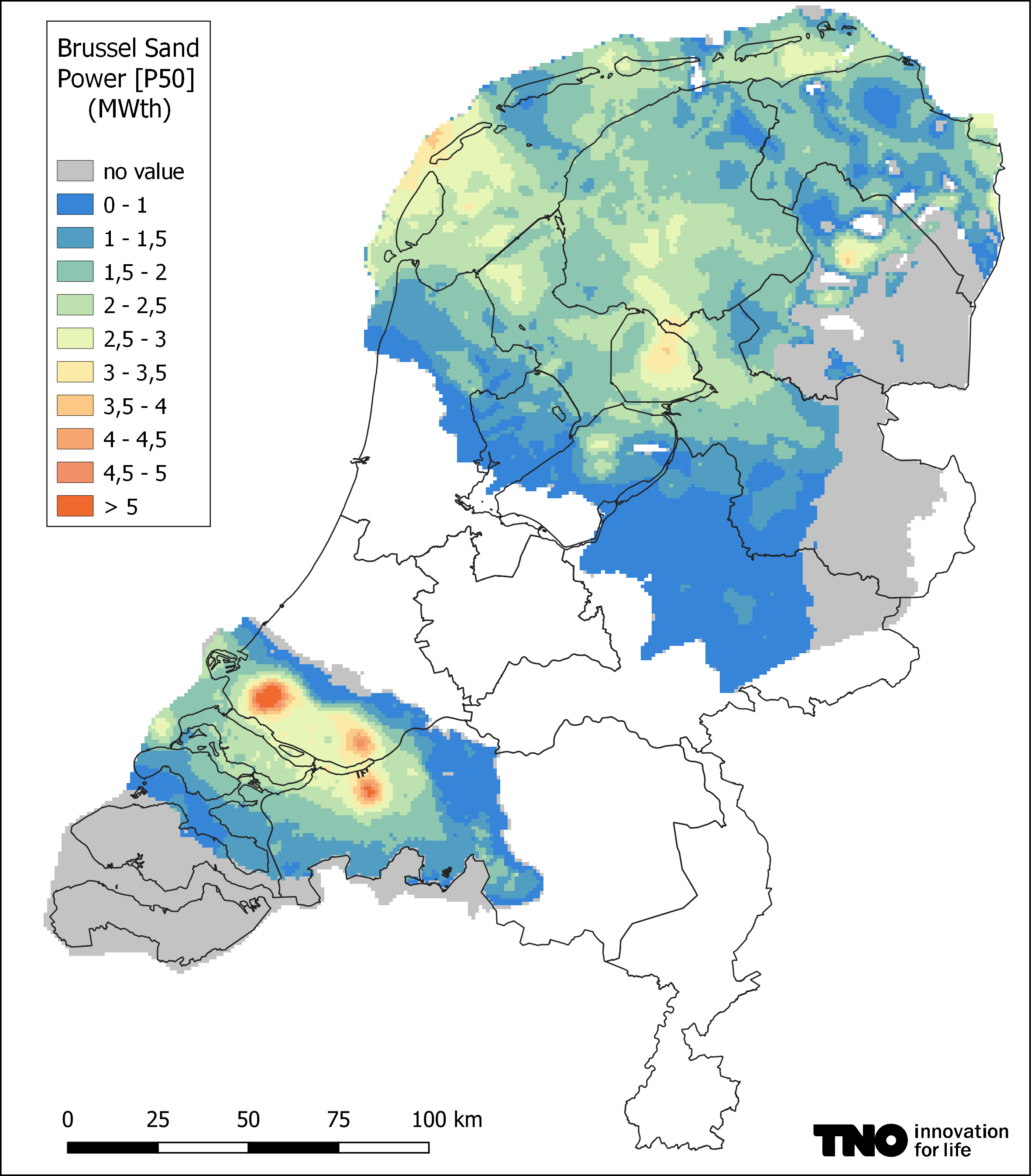d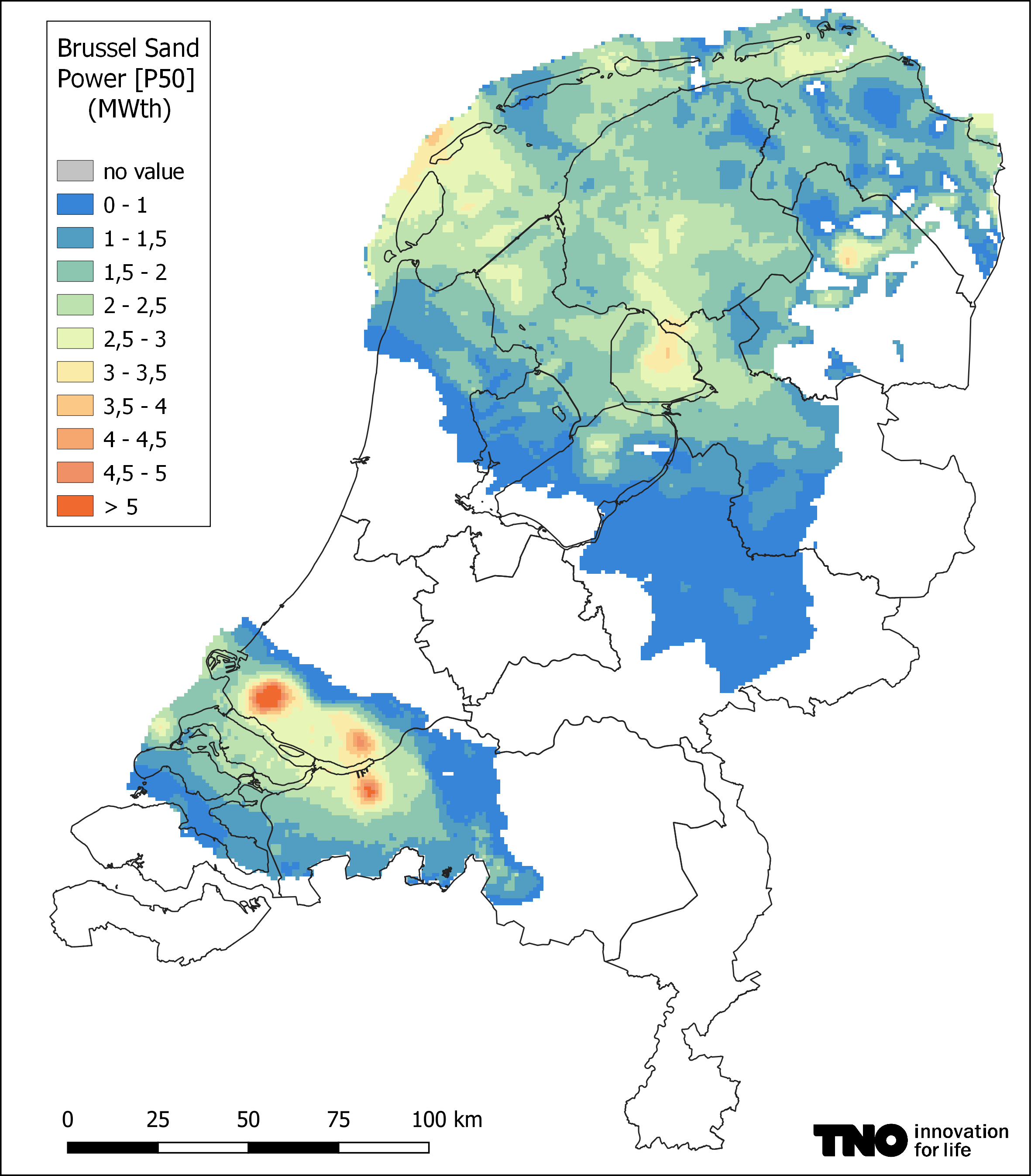Figure 3. Brussels Sand a) transmissivity; and power values calculated b) without step-out factor and without updated heat pump concept (standard ThermoGIS method with a constant COP of 4), c) with updated heat pump concept (COP calculated based on heat pump input and output), and d) with updated heat pump concept and a step out-factor of 2.